VI. Model Related Differential Equations: The Transcendental Functions. (Web text in progress.)
Preface: The definitions of polynomial and rational functions are easy to understand. They use only the arithmetic operations  learned in elementary school: addition, subtraction, multiplication, and division. The definitions of the exponential, logarithmic, and trigonometric functions are more advanced and sophisticated requiring an understanding of powers, roots, and geometry for similar triangles. What makes these latter functions important today is the fact that they appear in many different modelling contexts. One way in which these functions arise in models is through the study of differential equations. The following chart presents a summary of derivative results for the core functions we have studied together with their related characteristic differential equations with initial conditions.
 Function y =  f (x) Derivative y' = f ' (x ) Differential equation F(x, y, y', y'') = 0 Initial Conditions y(a) = b,... xn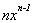xy' - ny = 0 y(0) = 0 sin(x) cos(x) y'' + y = 0 y(0) = 0 ; y'(0) = 1 cos(x) -sin(x) y'' + y = 0 y(0) = 1 ; y'(0) = 0 tan(x)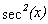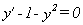y(0) = 0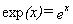exp(x) = ex y'-y = 0 y(0) = 1 ln(x) ... x>0 1/x xy'- 1 = 0 y(1) = 0

Looking at the differential equations in this table notice that power functions have characteristic differential equations that are more complicated than those of the other functions on the table. In this chapter we will explore further how differential equations are connected to the functions that solve them. To connect these functions to differential equations more precisely, we will start the first sections of this chapter by describing some modelling contexts. With each model we will consider a differential equation defining a solution function for that model. Studying these solution functions  we will recognize them as  familiar functions from properties and issues connected to the differential equaitions.

VI.A. The Natural Exponential Function and A Growth Model.

We begin our study models and differential equations with exponential functions. First we'll examine a model for populations that is based on a simple discrete difference equation. Then we will turn our attention to a comparable continuous calculus model. In our population model we assume we have a population of biological objects [they may be fruit flies, bacteria, rabbits, owls, fish, trees, and /or even humans], and this population is changing with time so that the measure of the population size, P, is a function of time, t. Note that P(t) may be measured with integers only, as with a population counter, or it may be measured with real numbers, as with biomass for bacteria, tonnage for fish, or aggregate height for trees.

 n P 0 * 1 ** 2 * * * * 3 ** ** ** **
Example VI.A.1. In a laboratory experiment a single cell breaks into two distinct cells every 8 hours. Starting with a single cell, this process continues for 40 hours in the same fashion.
• How many cells will be present at the end of the time period?
• If the process continues without change for t = 8n hours, how many cells will be present at time t?
• Estimate how long it will be before there are over 1000 cells.

•
Solution: Let P(n) be the number of cells present after 8n hours. Then P(0) = 1 expresses the statement that the experiment starts with a single cell and P(n+1) = 2 P(n) expresses the statement that every eight hours the number of cells doubles. Since 40 = 8 . 5, the first question asks us to find the value of P(5). Following routine calculations we have that P(1) = 2, P(2) = 4, P(3) = 8, P(4) = 16 and P(5)=32. So if the growth continues as stated in the problem, 32 cells will be present after 40 hours.
It is not hard to see from the pattern in these calculations that
P(n) = 2 n when t = 8n.

The last question can be rephrased, "For what n is 2n >1000?"
Here a quick check on the powers of 2 shows that 210  = 1024, so n = 10 is the smallest n that will satisfy the inequality. So P(10) = 210 >1000 shows we will need to wait about t = 8 * 10 = 80 hours, a little more than 3 days, for there to be 1000 cells.

Comment: In the example, the change in populations between 8 hour periods is not difficult to compute. If we let DP(n) denote the change in population expressed by the formula P(n+1)- P(n) then we can observe from the previous computations that P(0) = 1, P(1) = 2, P(2) = 4 , and
DP(n) = P(n+1)- P(n) = 2 n+1 - 2 n = 2 n = P(n).

The preceding example illustrates a model in which the population changes occur "discretely" in the sense that it is possible to isolate particular instants in time when the changes occur. The change in population,  DP(n), from the n th period to the (n+1) st period is determined precisely to be the value of the population at the end of the n th period.

The differential equation model: We now turn our attention to a model for populations in which the population measure changes as a continuous function of time. Assume a biological population for which at time t the population grows at a rate proportional to the population at that time. The growth rate of the population is expressed by the differential equation

P'(t) = k P(t) or dP/dt = kP,
with k being the proportionality constant. When t = 0  the population is P(0), the initial population.

Model units: We have some flexibility in the choice of the population unit of measurement in this model, so we will standardize our population measurement unit so that P(0) = 1. You can think of this standardization as measuring the size of the population when our observation begins and comparing subsequent populations in terms of that initial population. In other words, the populations are measured using the initial population as the unit for scaling.

The population growth rate, P'(t), is expressed in terms of population units per unit time. Since the unit of time is not yet determined for our model, we can choose a measurement for time so that the proportionality constant k is 1. Following this judicious choice of units we can now study more thoroughly the simpler model differential equation: P'(t) = P(t) .[See the comments at the end of this section  for a further exploration of the effects of choosing units.]

In summary then, the population size in our model is characterized mathematically as a particular solution to the differential equation P'(t) = P(t) with the initial condition P(0) = 1.

Visualization: Using the ideas of tangent fields from Chapter IV.D, we begin to understand the differential equation P'(t) = P(t) by sketching its tangent field.[See Figure 1.]By focusing attention on the initial condition, P(0)=1, we obtain a rough sketch of the graph of the solution function as an integral curve for this field.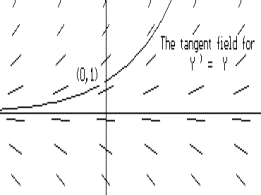The integral curve sketch confirms some information which the derivative gives us, namely, that P is increasing when P(t)>0 and that the graph of P is concave up [since P"(t) = P'(t) = P(t)] when P(t)>0. This information allows us to conclude further that the population in this model is increasing at ever increasing rates.

Estimating P(1): One immediate issue for this model is to learn the value of P(1). This will tell us the size of growth from one unit of population over one unit of time. We start to estimate P(1) using Euler's method with n = 4, so Dt = 1/4 and dP(t) = P'(t) Dt  = P(t) 1/4. Here are our calculations in a table:

 x P P ' = P dP = .25P 0 1 1 0.25 0.25 1.25 1.25 0.3125 0.5 1.5625 1.5625 0.390625 0.75 1.953125 1.953125 0.48828125 1 2.44140625

You can work with this further yourself using a speadsheet.
It is also worth looking at these calculations with a little more subtle technique, factoring as we go:
`P(0) = 1`
`P(1/4) ~~ P(0)+ P'(0) * 1/4 = 1 + 1 . 1/4 = 5/4`
`P(2/4)~~ P(1/4) + P'(1/4)*1/4 ~~ 5/4 + 5/4 * 1/4 = (5/4) 2`
`P(3/4) ~~ P(2/4)+P'(2/4) *1/4 ~~ (5/4) 2+(5/4) 2 * 1/4=(5/4) 3`
`P(1) ~~ (3/4) + P'(3/4) *1/4~~ (5/4) 4 ~~ 2.4414`
Okay. So our first estimate using Euler's method with n = 4 is that `P(1) ~~ 2.4414`. But we can do better than that, much better.
Note that since `P'(t) = P(t),  P(a + dt)~~ P(a) + P(a) dt = P(a)(1 + dt)` .
If we use  `dt = h = 1/n` for `n`, a positive integer, then `P(1/n)~~ 1 + 1 . 1/n`,  `P(2/n)~~ P(1/n) + P'(1/n) . 1/n = (1+1/n)^2` and continuing in this fashion we obtain
`P(1) = P(n/n)~~ (1+1/n)^ n.`
For `n` very large,   `dt = h = 1/n` will be close to 0 and we should obtain a much better approximation for `P(1)` from Euler's method. In limit notation we write
`lim_{n to oo} (1+1/n)^n = lim_{h to 0} (1+h)^{1/h} = P(1)`.

Using this estimation with `n = 1000`, we obtain `P(1)~~(1+.001)^{1000} ~~  2.7169` and with `n = 10,000`, we find `P(1)~~(1+.0001)^10000 ~~  2.7181`. With `n = 10^6, P(1)~~ (1+10^{-6})^{1,000,000} ~~ 2.718280469`.
[In fact, the convexity of `P` helps explain why in theory these are all underestimates. Can you see why?]
You may recognize from these estimates that the exact value of `P(1)` is a number studied in your pre-calculus work. It is a mathematical constant which has been of interest for hundreds of years and, like the number `pi`, has a special symbol attached to it, `e` . [Actually the letter `e` was first used to represent this constant extensively by Euler, whose textual treatment of both pre-calculus and calculus topics were the standard for many years.] Thus, by notational convention and our previous estimates we have that `P(1) = e~~ 2.718` .

Properties of P: We turn now to explore some of the important features of `P` as a function.
If it takes one unit of time for one unit of population to grow to be e units of population, you might expect that in one unit of time any population size will grow by a factor of e.

Conjecture: For any `t, P(t+1) = P(1)*P(t)`
Comment: We can make more sense of this conjecture from our previous use of Euler's method:
Since `P(t + dt)~~ P(t) + P(t) dt = P(t)(1+dt)` so `P(t + n *dt)~~P(t)(1+ dt)^n`.
With `dt=1/n` this shows that `P(t+1) = P(t+ n* 1/n)~~P(t)*(1+1/n)^n`.
When `n` is large we can see why `P(t+1) = P(t)*P(1)`.

Proof of Conjecture: We can prove that the conjecture is true for all t in a more rigorous fashion by considering the function F(t) = P(t+1)/P(t) .

Using the quotient and Chain Rules we find F '(t) .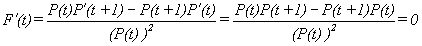.
So [by Theorem IV.**,] F(t) is a constant function, i.e., F(t) = K for all t.
But using t = 0, we see that F(0) = P(0+1)/P(0) = P(1)/P(0) = e/1 = e.So,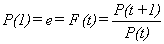for all t, or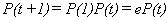.
EOP.
Comment: Note how this equation was proven. First we examined a related function Fand showed that F was a constant function because F '(t) = 0. Then we used the particular value of F when t = 0 to find the constant, allowing us then to prove the original equation was true.

From the conjectured and now proven equation we see that

P(2) = P(1+1) = P(1) . P(1) = e . e = e 2;
P(3) = P(2+1) = P(1) . P(2) = e . e 2 = e 3;
and in general  we can show (using mathematical induction) that for any natural number n,
P(n) = e n .

It appears that P is the exponential function with base e. We proceed now to justify that P satisfies the usual properties of exponents using only its characterization from the differential equation. [These results are also suggested by further exploration of Euler's approximations for the values P(n) and the definition of e. See Exercise 14.]

Theorem VI.A.1 Suppose s and t are real numbers and P is the solution to the differential equation P'(t) = P(t) with P(0) = 1. Then

(a) P(s+t) = P(s) . P(t),

(b) P(-t) = 1/P(t),

(c) P(s-t) = P(s)/P(t), and

(d) P(rt)=[P(t)]r when r is an rational number.

PROOF: (a) We proceed as we did in justifying the previous conjecture.
Let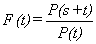thenso F(t) = K for some constant K.
But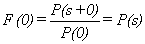.
Hence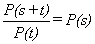or P(s+t) = P(s) . P(t) .
(b) P(t+(-t)) = P(0) = 1 but by (a) P(t+(-t)) = P(t)P(-t) so 1 = P(t) . P(-t) OR  P(-t) = 1/P(t).
(c) P(s-t) = P(s+(-t)) = P(s) . P(-t) = P(s) . 1/P(t) = P(s)/P(t).
(d) Consider F(t) = [P(t)] r/P(rt) then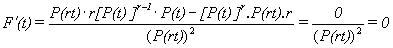.

so F(t) = k for some constant k.

But F(0)=[P(0)] r/P(r.0)=1r/1=1 so 1=[P(t))] r/P(rt)or P(rt) = [P(t)] r.
EOP.

 Defining the natural exponential function: We could give a definition for the natural exponential function characterizing it solely as the solution to the differential equation y'=y where y(0)=1. Defining a function by declaring it to be the unique solution to a differential equation is not as unusual as it may seem. Recall that in the motion interpretation for the derivative a differential equation can be interpreted as giving a way to determine the velocity of a moving object from the time and its position. Knowing the velocity of the object at any time and position would seem to enable us to determine its position at any time just from knowing its position at one time.
1. P(t)=e t : Applying the last part of the theorem to an rational number r, we see that P(r) = P(1) r = e r. Since P is a continuous function, this shows that P has the same values as the exponential function with base e for any real number t, so we can write P(t)=e t for any real number t. Recognizing this identification of P with the exponential function of base e, we express the key facts we have demonstrated about P using the Liebniz notation for the derivative and the exponential notation as follows: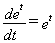; e 0=1; and e 1 =e.
Also
(a) e s+t = e s.e t;
(b) e -t =1/e t;
(c) e s-t = e s/e t;
(d) e r t = (e t) r.

2.Asymptotes: Note that since e > 2 , e 2 > 2 2 , e 3 > 2 3and generally e n > 2 n. So when n is very large, e n is very large as well. This fact is expressed as a limit statement by writing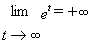.
Also, since e -n = 1/e n , when n is very large , e -n is the reciprocal of a large number.  Thus for large choices of n, e -n  » 0. This is expressed as a limit statement by writing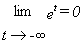.
On the graph of P(t) = e t this last fact is recognized by the horizontal asymptote of the graph with the X - axis.
3. Looking at integration: Sincewe can write the indefinite integral form of this statement as.
4. Existence: The preceding discussion assumed that there was a solution to the differential equation y' = y with y(0) = 1. The existence of a solution is certainly suggested by the tangent field and the computations using Euler's method. A rigorous demonstration of the existence of this function independent of any assumptions about exponential functions and their properties will be given later in this chapter. For now, all the evidence we have assembled suggesting the conclusion should suffice.
5.Other models: The general issue of population models and differential equations is studied in greater depth in more advanced courses in differential equations and mathematical modeling. The differential equation y' = ky with initial condition y(0) = A is very important to the study of numerous other real situations besides this simple population growth model. In exercise 8 below you are asked to check that the general solution of this differential equation is y = A e kt .

6. Changing units to normalize the model: [This next comment is pretty tricky. Be sure to read this with a pencil and paper at hand to make sure you follow the changes in the units.]
As suggested initially, a suitable choice of units shows the relation of the special case when k = 1 and A = 1 to the general situation with y'(t)=ky(t) and y(0) = A.

Here's how: Suppose the units for y are called yugs and the time t is measured in tocks.We'll let A yugs be our new unit for measuring the population with the variable z, which we'll call zugs, so A yugs is one zug. We'll let 1 / k tocks be our new time units, we'll call them nocks, and we'll uses u to measure the time in nocks, so k nocks is one tock. So y = A z and t =u.

Now what is the relation between the measure of the population measure as y yugs at time t tocks, y(t), and the measure of the same population measure as z zugs at the same time but measured as u nocks, z(u)? Well, Az(u) expresses the measure of the population in yugs based on its measurement in mugs,while y() expresses the measurement of the population at the same time measured in tocks also in the units of yugs. So Az(u) = y(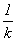u). Now we suppose that y(0)=A, so Az(0)=y(0)=A and z(0)=1. Furthermore since z(u) = 1/ A y(u) and we assume y'(t)= ky(t), we have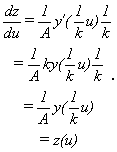So if y satisfies the original differential equation y '= ky then z satisfies the differential equation z' = z with z(0) = 1.But our work has shown then that z(u) = e u. Now translate this back into a statement about y:
Az(u) = y(u) whereu = t.
So y(t) = A z( kt ) = A e kt.

7. Other Applications of y'=ky with y(0) = A
There are many other situations where the differential equation beginning this section arises in expressing the rate of change of a measured quantity. One important application is to the measurement of the amount of radioactive material present in a given mass of material. Because of the nature of unstable radioactive matter, the rate of change (decay) of the matter is assumed to be proportional to the amount of matter present at the specified time. This should make some sense from a physical point of view. With less unstable material present there is less opportunity for the interaction of unstable particles to produce further radioactive decay.

Another situation involving this basic differential equation concerns the change in temperature of one object in a medium which remains at a constant temperature. In this context we find a physical context to which one of Newton's laws, called his law of cooling, applies. The law says that the rate of change of the temperature is proportional to the difference in temperatures of the object and the medium.These situation will be explored further in the exercises of section VI.D.
The exercises for this section apply many of the concepts and techniques we have developed already in the earlier chapters to a further exploration of the exponential function and functions that use the exponential function in their definition.
Exercises VI.A.:
1. Find the derivatives of the functions as indicated. [Don't forget the chain rule!]
a. f(x) = exp ( 5x ) . Find f '(0) , f '(1) and f '(t).
b. f(x) = exp ( -x ) . Find f '(0) , f '(1) and f'(t).
c. f(x) = exp ( x 2) . Find f '(0) , f '(1) and f'(t).
d. f(x) = exp (-x 2) . Find f '(0) , f '(1) and f'(t).
e. f(x) = exp (sin(x)). Find f '(0) , f '(p) and f '(t).
2. Find the derivatives of the functions as indicated. [Don't forget the chain rule!]
a. y = e 3x . Find dy/dx when x = 0 and 1.
b. y = x e x. Find dy/dx when x = 0 and 1.
c. y = e x sin(x). Find dy/dx.
d. y = e x +e -x. Find dy/dx when x = 0.
e. y = e x -e -x. Find dy/dx when x = 0.
3. Find the derivatives of the functions as indicated. [Don't forget the chain rule!]
a. Find D t(sin(e t)).
b. Find D t(exp(t 2 - 1)).
c. Find D t( exp(t sin(t) ) ).
d. Find D t(e t / t 2).
e. Find D t(sec(e 2t ) ).
4. Sketch a graph of y = exp(-x 2 / 2) showing all extrema and points of inflection. Explain your work using first and second derivative analysis.
5. Sketch a graph of y = x e -x showing all extrema and points of inflection. Explain your work using first and second derivative analysis.
6. Sketch a graph of y = x 2 e -x showing all extrema and points of inflection. Explain your work using first and second derivative analysis.
7. Sketch a graph of y = sin(x) e -xfor x e [0,4p], showing all extrema and points of inflection. Explain your work using first and second derivative analysis.
8. Show that y = A e kx is a solution to the differential equation y'=ky with y(0) = A.
9. Show that y = e x (sin(x)+cos(x)) is a solution to the differential equation y' = 2 e xcos(x) and y'' - y' = -2e xsin(x).[Corrected 9-9-02 4:30 pm ]
10. Show that y=e x(sin(x)+cos(x)) is a solution to the differential equation y''-2y' +2y = 0.
11. Suppose f(x) = k e x is the probability density function for a random variable X on the interval [0,1]. Show that k = 1/(e-1).
12. Suppose f(x) = k e -x is the probability density function for a random variable X on the interval [0,1]. Find k .
13. Find the following indefinite integrals:

 a. ò e3x dx c. ò cos(x) esin(x) dx b.ò x exp(x2 )dx d. ò ex sin(ex )dx
14. Use Euler's method to explain why when n >> 0, (1+1/n) 2n » e 2, (1+1/n) 3n »e 3,
(1+1/n) 4n » e 4, and in general (1 + 1/n) kn » e k.
15. Find the following definite integrals. You may express your answer using the number e.
a.   ò01 e3x dx                      b. ò01 x exp(x2 )dx
16. Find the area of the region enclosed by the graph of Y= e x = exp(X), the X axis, X= -1, and X= 1.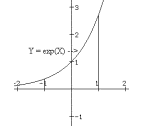17. The area of the region enclosed by the graph of, the X axis, X= -1, and X= 1 is often of interest in the study of probability and statistics. Estimate this area using Euler sums with a. n=4. b. n=8. c. n = 100.
18. The region in the plane bounded by the graph of, the X-axis, the Y axis, and the line X=1 is rotated about the Y- axis to form a region in space.Find the volume of this spatial region.
19. The region in the plane bounded by the graph of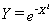, the X-axis, the Y-axis, and the line X= t is rotated about the Y- axis to form a region in space.Find the volume of this spatial region.Give an estimate for the volume of the this region in space when t = 100.

 This confirms what we already might have guessed from our previous knowledge from Chapter *** about the derivative of this exponential function since the function ex does satisfy the differential equation with the given initial condition. What is of interest here is that we have arrived at this connection starting with a model for population growth and a differential equation without presuming what the solution would be.